# Uniswap v3 详解（一）：设计原理

Posted on March 28, 2021

## 设计原理

Uniswap v2 版本使用 $x \cdot y = k$ 这样一个简洁的公式实现了 AMM Dex，正是由于其简洁易用性，使其在短短的一年的时间内迅速成长为 DeFi 领域的龙头项目。但是随着 DeFi 生态走过了「从无到有」的阶段，因为 v2 无法满足某些特定需求，从而诞生了 Curve, Balancer 这些针对某些功能进行改进的 AMM。

• 可灵活选择价格区间提供流动性
• 更好用的预言机
• order book 功能
• 灵活的费率

### v2 的问题

$k = 4500 \times 3 = 13500$

$\begin{cases} x_2 \cdot y_2 = 13500 \\ \displaystyle \frac {x_2}{y_2} = 1300 \end{cases}$

### 解决方案

v3 版本的解决方案是允许用户只在一段价格区间内提供流动性。如下图：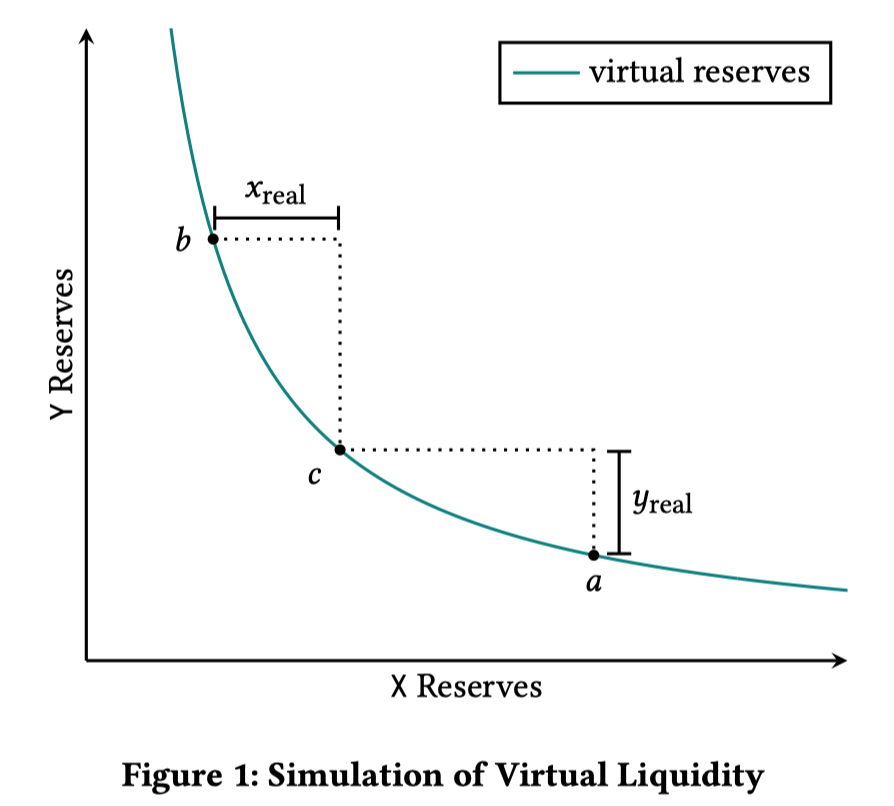$x = x_{virtual} + x_{real} \\ y = y_{virtual} + y_{real}$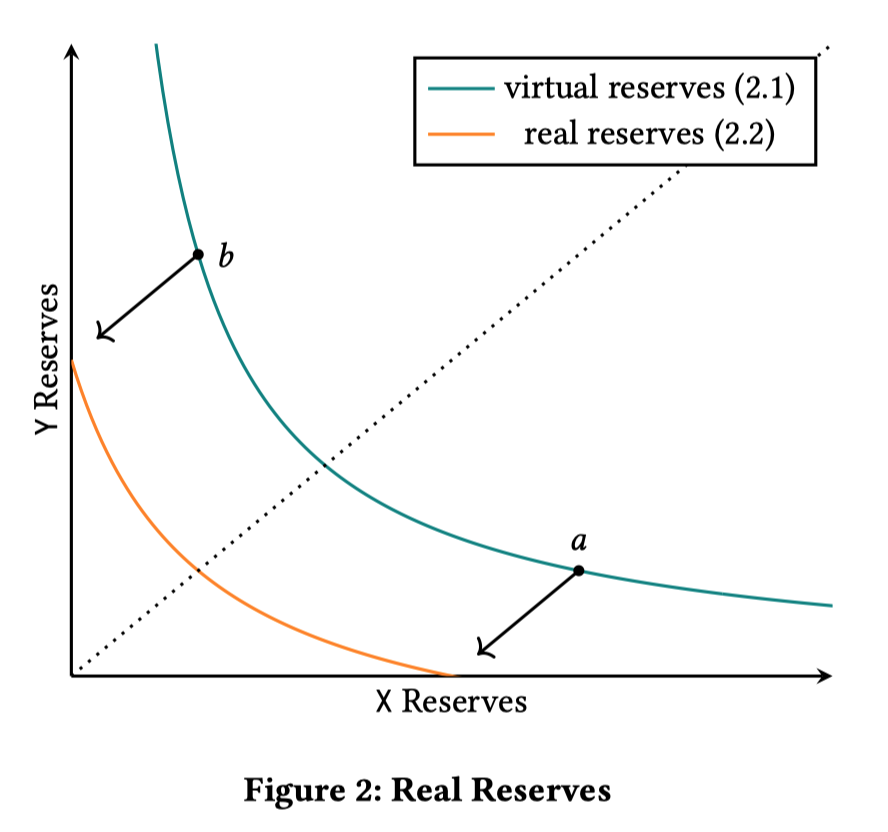### 交易过程

#### v2 版本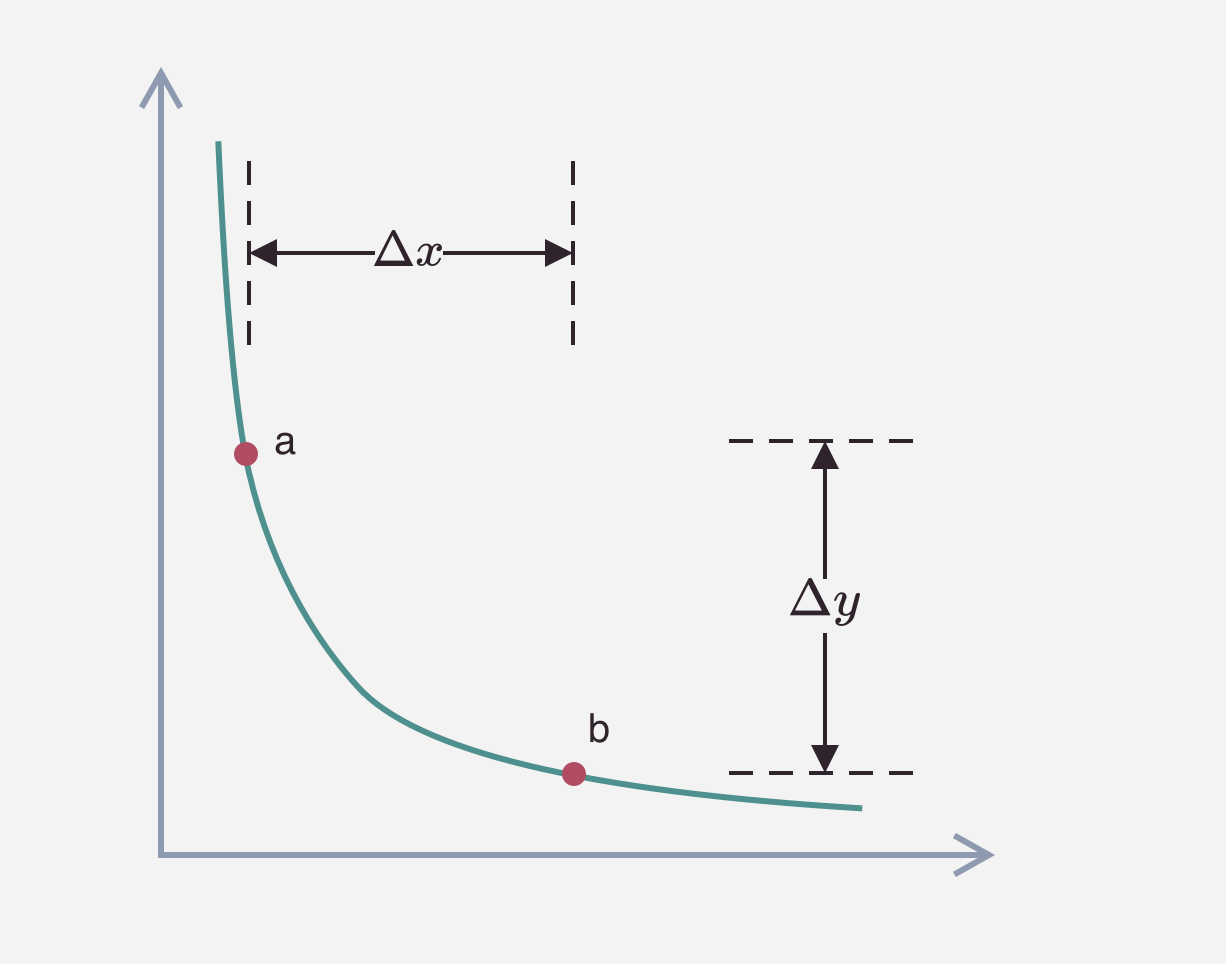$x \cdot y = (x + \Delta x)(y - \Delta y) = k$

$\Delta y = y - \frac {x \cdot y}{x+\Delta x} = \frac {\Delta x y}{x + \Delta x}$

#### v3 版本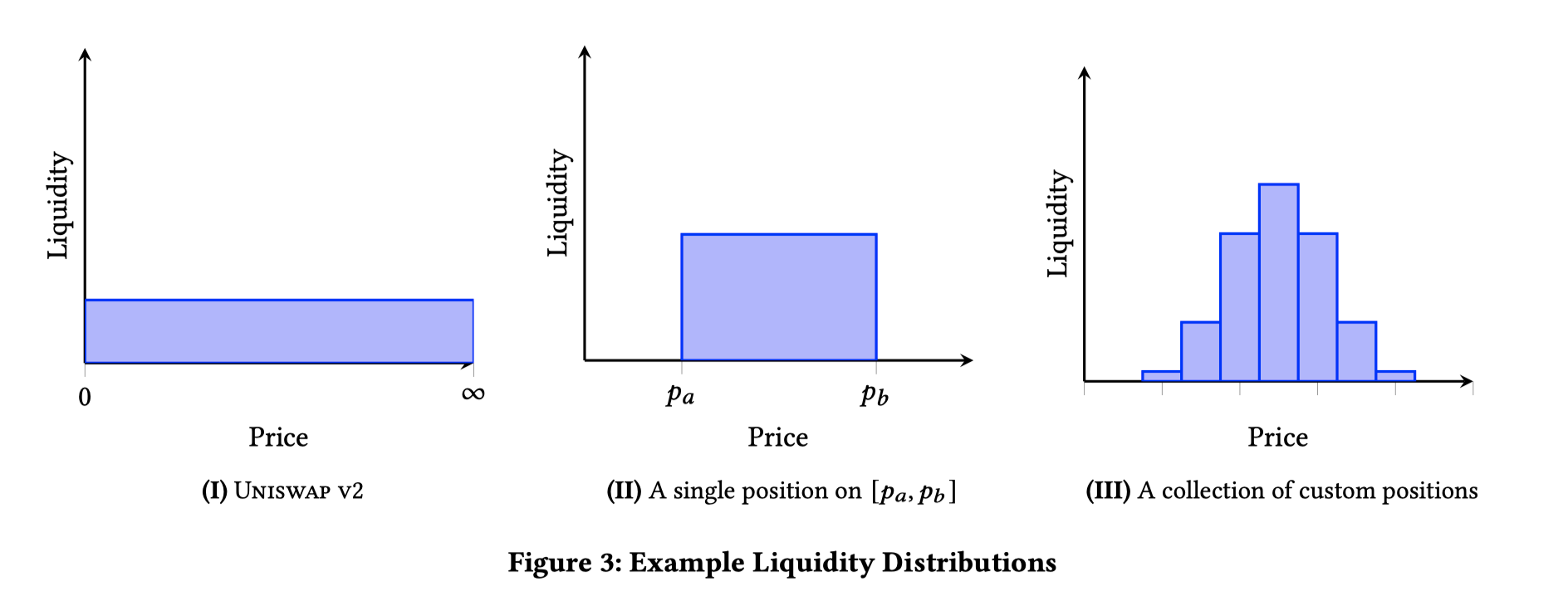• 交易至指定价格（不可以超出此流动性的边界价格）$P$，需要的 x token 数 $\Delta x$，可以获得的 y token 数 $\Delta y$
• 给定 x token 数 $\Delta x$（假设不会引发价格超出此流动性的边界价格），可以获得的 y token 数 $\Delta y$，以及最终的价格 $P$

$\begin{cases} x \cdot y = k \\ P = \displaystyle \frac {y}{x} \end{cases}$

$\begin{cases} x = \sqrt \frac {k}{P}} \\ y = \sqrt {kP} \end{cases} \Rightarrow \begin{cases} \Delta x = \Delta \displaystyle \frac 1{\sqrt P} \cdot \sqrt k \\ \Delta y = \Delta \sqrt P \cdot \sqrt k \end{cases$

### 价格精度问题

• 几乎很难有两个流动性设置相同的价格边界，这样会导致消耗大量合约存储空间保存这些状态
• 当进行交易计算时，价格变化被切分成很多个小的范围区间，需要逐一分段进行计算，这会消耗大量的 gas，并且如果范围的价差太小，可能会引发计算精度的问题

Uniswap v3 解决这个问题的方式是，将 $[P_{min}, P_{max}]$ 这一段连续的价格范围为，分割成有限个离散的价格点。每一个价格对应一个 tick，用户在设置流动性的价格区间时，只能选择这些离散的价格点中的某一个作为流动性的边界价格。

Uniswap v3 采用了等比数列的形式确定价格数列，公比为 1.0001。即下一个价格点为当前价格点的 100.01%，前面我们说过 Uniswap v3 实际存储的是 $\sqrt P$，那么下一个价格与当前价格的关系为

$\sqrt {P_{next}} = \sqrt {1.0001} \cdot \sqrt {P_{current}}$

### tick 管理

$i = \log_{\sqrt {1.0001}}{\sqrt p}$

Uniswap 不需要记录每个 tick 所有的信息，只需要记录所有作为 upper/lower tick 所包含的流动性元数据即可。看下面这个例子：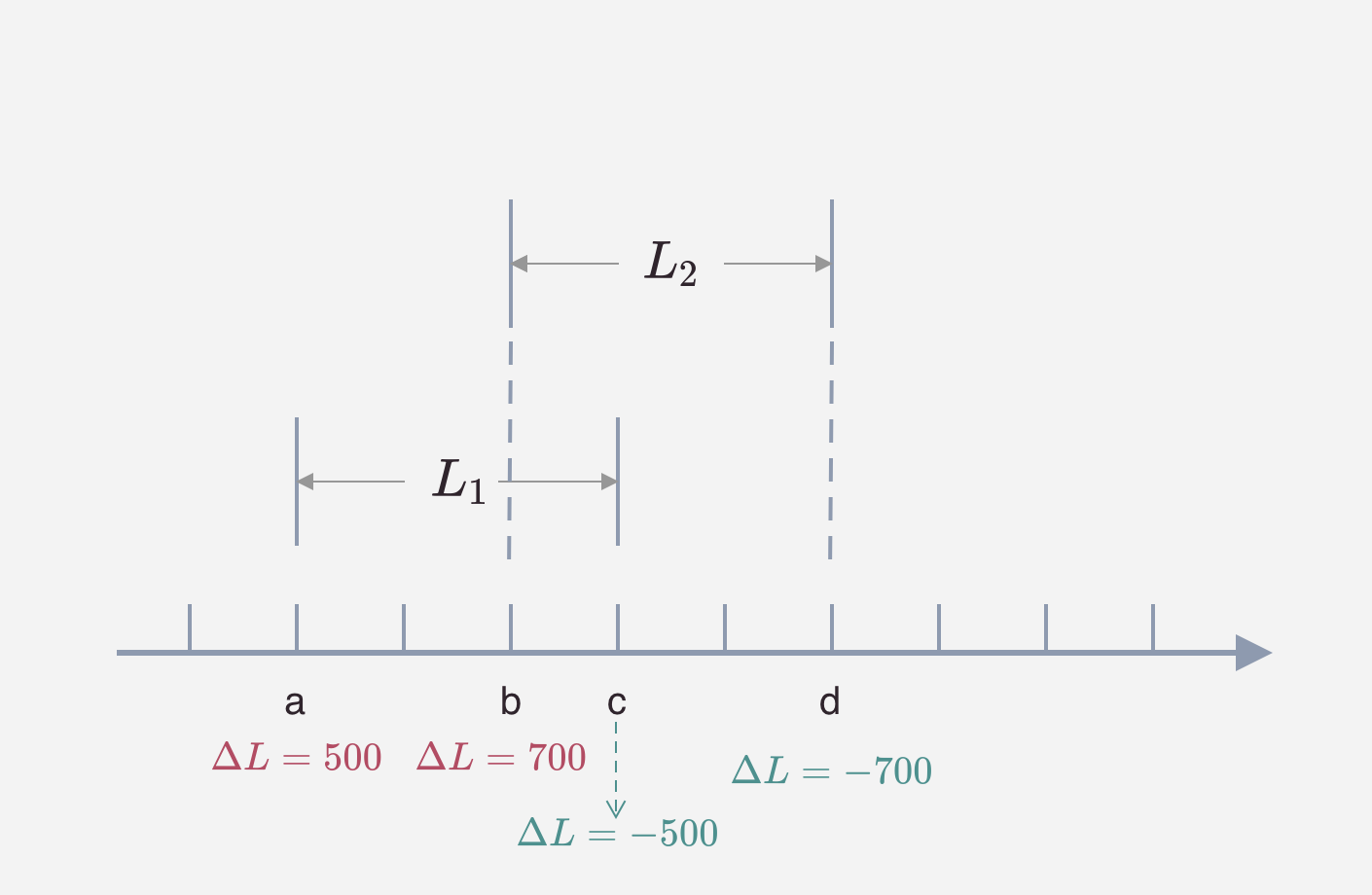### 灵活的手续费选择

v3 版本内置了三种梯度的手续费率（0.05%, 0.30%, and 1.00%），同时可以在未来增加更多的费率值。关于手续费的计算过程，这部分放在后文来详解（链接：交易手续费）。需要注意的是，由于需要支持多种费率，同一个代币对 v3 版本会有多个不同的流动池。例如 ETH/DAI 代币对，会分成三个池，分别对应 0.05%, 0.30%, 1.00% 的手续费。

#### 手续费与 tick 的关系

Uniswap 默认设置了费率和 tickSpacing 的关系：

0.05% 10
0.30% 60
1.00% 200

## 代码架构

Uniswap v3 在代码层面的架构和 v2 基本保持一致，将合约分成了两个仓库：

core 仓库的功能主要包含在以下 2 个合约中：

• UniswapV3Factory: 提供创建 pool 的接口，并且追踪所有的 pool
• UniswapV3Pool: 实现代币交易，流动性管理，交易手续费的收取，oracle 数据管理。接口的实现粒度比较低，不适合普通用户使用，错误的调用其中的接口可能会造成经济上的损失。

peirphery 仓库的功能主要包含在以下 2 个合约：

• SwapRouter: 提供代币交易的接口，它是对 UniswapV3Pool 合约中交易相关接口的进一步封装，前端界面主要与这个合约来进行对接。
• NonfungiblePositionManager: 用来增加/移除/修改 Pool 的流动性，并且通过 NFT token 将流动性代币化。使用 ERC721 token（v2 使用的是 ERC20）的原因是同一个池的多个流动性并不能等价替换（v3 的集中流性动功能）。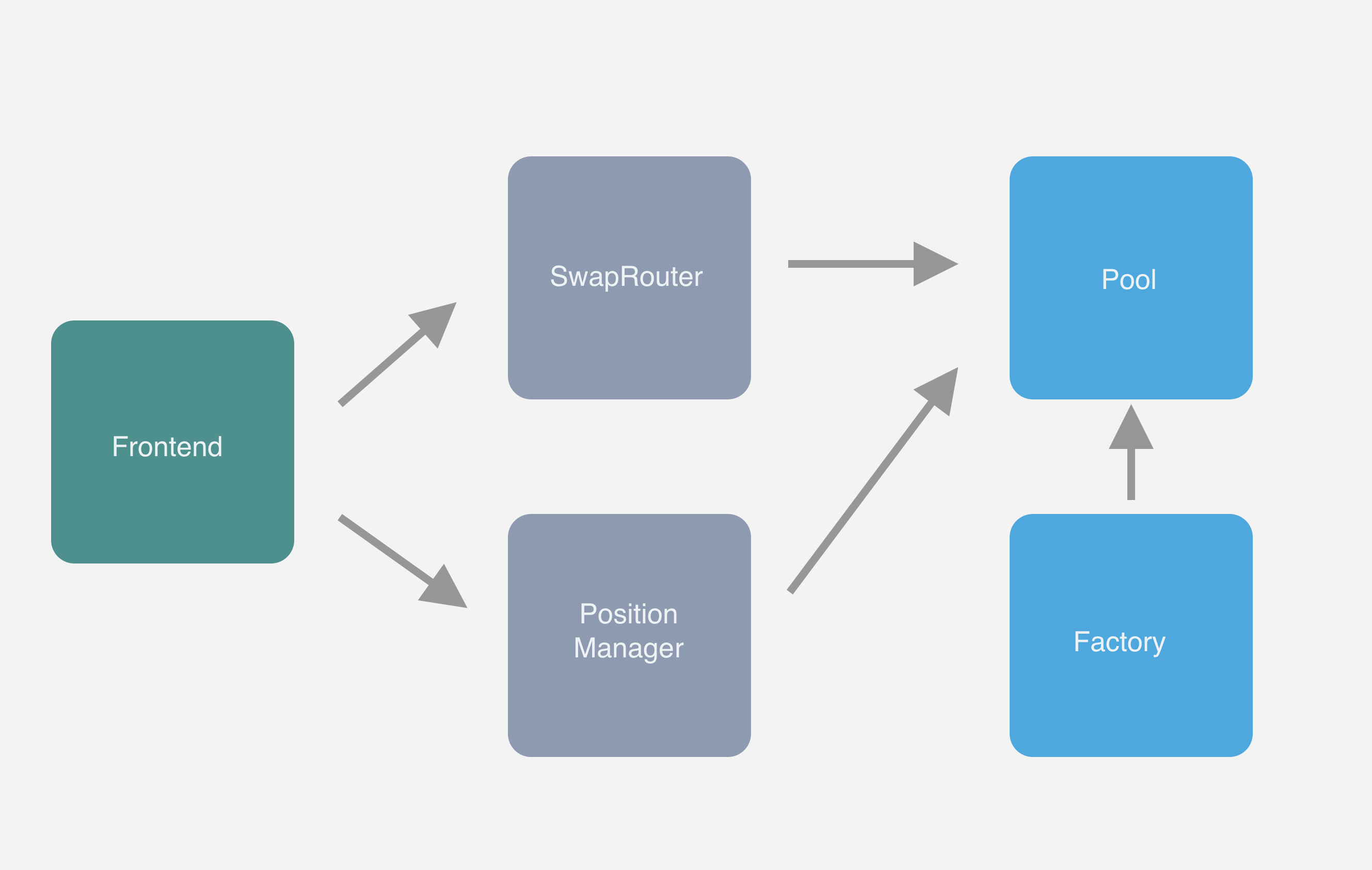## Uniswap v3 详解系列